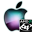ATOMS : Mathieu functions toolbox details

# Mathieu functions toolbox

Solve Mathieu equations
Details
Version
2.0
A more recent valid version with binaries for Scilab 5.2 exists: 3.0
Authors
R. Coisson, G. Vernizzi, XK. Yang, N. Strelkov
Michael Baudin
Owner Organization
Parma University - DIGITEO
Maintainers
Michael Baudin
Robert Coisson
Nikolay Strelkov
Category
Dependency
Creation Date
June 8, 2011
Source created on
Scilab 5.2.x
Binaries available on
Scilab 5.2.x:Windows 64-bitWindows 32-bitLinux 64-bitLinux 32-bitmacOS
Install command
`--> atomsInstall("Mathieu")`
Report a bugDescription
```            This Mathieu Functions Toolbox is used to solve Mathieu function numerically.

The Mathieu equation is a second-order homogeneous linear differential equation

and appears in several different situations in Physics: electromagnetic or
elastic
wave equations with elliptical boundary conditions as in waveguides
or resonators, the motion of particles in alternated-gradient
focussing or electromagnetic traps, the inverted pendulum,
parametric oscillators, the motion of a quantum particle in
a periodic potential, the eigenfunctions of the quantum pendulum, are just few
examples.
Their solutions, known as Mathieu functions,
were first discussed by Mathieu in 1868 in the context of the
free oscillations of an elliptic membrane.

The toolbox has 8 demos.
All demos can be acessed from "?->Scilab Demonstrations"

Features
--------
* mathieu_cart2ell : Converts from cartesian to elliptical coordinates.
* mathieu_ell2cart : Converts from elliptical to cartesian coordinates.
* mathieu_ellipse : 3D plot of a mode with elliptical boundary conditions.
* mathieu_elliptical : 3D plot of a mode with elliptical boundary conditions.
* mathieu_expqa : Plot nu with respect to q and a.
* mathieu_mathieu: Evaluates periodic Mathieu functions.
* mathieu_mathieuS: Calculates solutions of Mathieu Equation.
* mathieu_mathieuexp:  Evaluates the characteristic exponent.
* mathieu_mathieuf: Evaluates characteristic values and expansion
coefficients.

Bibliography
------------
* R. Coïsson, G. Vernizzi and X.K. Yang, "Mathieu functions and numerical
solutions of the Mathieu equation", IEEE Proceedings of OSSC2009, online at http://www.fis.unipr.it/~coisson/Mathieu.pdf

* N.W. McLachlan, Theory and Application of Mathieu Functions, Oxford Univ.
Press, 1947
* J. C. Gutiérrez-Vega, R. M. Rodríguez-Dagnino, M. A. Meneses-Nava, and S.
Chávez-Cerda, "Mathieu functions, a visual approach", American Journal of
Physics, 71 (233), 233-242. An introduction to applications, online at http://www.df.uba.ar/users/sgil/physics_paper_doc/papers_phys/modern/matheiu0.pdf
```Files (2)Source code archive
```
```OS-independent binary for Scilab 5.2.x
```Binary version
Automatically generated by the ATOMS compilation chain

```News (0)Comments (2)
[get_person] Le compte avec l'identifiant 2125 n'existe pas